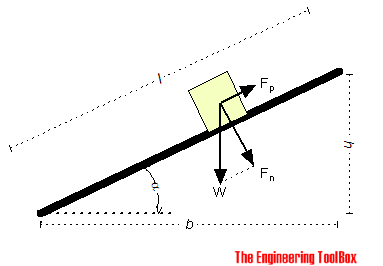Engineering ToolBox - Resources, Tools and Basic Information for Engineering and Design of Technical Applications!

# Bodies Moving on Inclined Planes - Acting Forces

## Required forces to move bodies up inclined planes.If we neglect friction between the body and the plane - the force required to move the body up an inclined plane can be calculated as

Fp = W h / l

= W sin α

= m ag sin α                            (1)

where

Fp = pulling force (N, lbf)

W = m ag

=  gravity force - or weight of body (N, lbf)

h = elevation (m, ft)

l = length (m, ft)

α = elevation angle (degrees)

m = mass of body (kg, slugs)

ag = acceleration of gravity  (9.81 m/s2, 32.174 ft/s2)

By adding friction - (1) can be modified to

Fp = W (sin α + μ cos α)

= m ag (sin α + μ cos α)                            (2)

where

μ = friction coefficient

### Example - Pulling Force on Inclined Plane

A body with mass 1000 kg is located on a 10 degrees inclined plane. The pulling force without friction can be calculated as

Fp = (1000 kg) (9.81 m/s2) sin(10°)

= 1703 N

= 1.7 kN

### Online Inclined Plane Force Calculator - SI Units

The calculator below can be used to calculate required pulling force to move a body up an inclined plane.

mass of body - m - (kg)

elevation angle - α - (degrees)

friction coefficient - μ - (zero when neglecting friction)

### Online Inclined Plane Force Calculator - Imperial Units

mass of body - m - (slugs)

elevation angle - α - (degrees)

friction coefficient - μ - (zero when neglecting friction)

### Angle of Repose

A body resting on a plane inclined at at an angle α to the horizontal plane is in a state of equilibrium when the gravitational force tending to slide the body down the inclined plane is balanced by an equal and opposite frictional force acting up the inclined plane.

For equilibrium the "angle of response" α can be expressed as:

μ = Fp / Fn = (W sin α) / (W cos α) = tan α                                   (3)

### Example - Gradient Force acting on a Car, Work Done and Power Required

The weight of a Tesla Model X is 2400 kg. The force acting on the car with 5% inclination can be calculated from (1) as

Fp_5% = (2400 kg) (9.81 m/s2) sin (5°)

= 2051 N

The force acting on the car with 10% inclination can be calculated as

Fp_10% = (2400 kg) (9.81 m/s2) sin (10°)

= 4088 N

If the Tesla is moving along the inclined roads with the same speed - the work done by the forces after 1 km can be calculated as

W5% = (2051 N) (1000 m)

= 2051 kJ

W10% = (4088 N) (1000 m)

= 4088 kJ

If the speed of the Tesla is 80 km/h (22.2 m/s) - the time to drive the distance can be calculated as

t80 = (1000 m) / (22.2 m/s)

= 45 s

The power required to move the vehicle (without rolling and air resistance) can be calculated as

P5% = (2051 kJ) / (45 s)

= 45.6 kW

P50% = (4088 kJ) / (45 s)

= 90.8 kW

If the speed of the car is reduced to 60 km/h (16.6 m/s) - the time to drive the distance can be calculated as

t60 = (1000 m) / (16.6 m/s)

= 60.2 s

The power required to move the vehicle (without rolling and air resistance) can be calculated as

P5% = (2051 kJ) / (60.2 s)

= 34.1 kW

P50% = (4088 kJ) / (60.2 s)

= 67.9 kW

## Related Topics

• Dynamics - Motion - velocity and acceleration, forces and torque.
• Mechanics - Forces, acceleration, displacement, vectors, motion, momentum, energy of objects and more.

## Engineering ToolBox - SketchUp Extension - Online 3D modeling!

Add standard and customized parametric components - like flange beams, lumbers, piping, stairs and more - to your Sketchup model with the Engineering ToolBox - SketchUp Extension - enabled for use with the amazing, fun and free SketchUp Make and SketchUp Pro .Add the Engineering ToolBox extension to your SketchUp from the SketchUp Pro Sketchup Extension Warehouse!

Translate

## Privacy

We don't collect information from our users. Only emails and answers are saved in our archive. Cookies are only used in the browser to improve user experience.

Some of our calculators and applications let you save application data to your local computer. These applications will - due to browser restrictions - send data between your browser and our server. We don't save this data.

## Citation

• Engineering ToolBox, (2008). Bodies Moving on Inclined Planes - Acting Forces. [online] Available at: https://www.engineeringtoolbox.com/inclined-planes-forces-d_1305.html [Accessed Day Mo. Year].

Modify access date.

. .

#### Scientific Online Calculator3 30